# ISEE Lower Level Math : Ratio and Proportion

## Example Questions

← Previous 1 3 4 5 6

### Example Question #1 : How To Find A Ratio

In order to make Lilly's Lemonade, for every 16 oz of water, 6 tablespoons of lemon juice is needed. If you want to use 8 oz of water, how much lemon juice would you need?

3 tablespoons

14 tablespoons

4 tablespoons

8 tablespoons

12 tablespoons

3 tablespoons

Explanation:

In order to solve this problem, we must set up a ratio.

For every 16 oz of water, we need 6 tablespoons of lemon juice.

The ratio that we get is

16 : 6.

If we want to use only 8 oz of water, we need to find out how much lemon juice is needed.

The amount of lemon juice we need, x, is currently unknown.

Our new ratio is

8 : x.

Looking at the first number in the two ratios, dividing 16 by 8 will get us

16 ÷ 8 = 2.

Let's divide the second number in our first ratio by 2 to solve for x.

So,

6 ÷ 2 = 3.

We need 3 tablespoons of lemon juice for 8 oz of water.

### Example Question #2 : How To Find A Ratio

Find the ratio

If there are 3 frogs at a pond and 12 toads, what is the ratio of frogs to toads at the pond?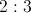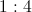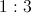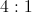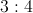Explanation:

Since there are 3 frogs and 12 toads, reduce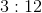by 3 to get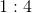.

### Example Question #3 : Ratio And Proportion

Janie has a bag full of red, blue, orange, white, and green marbles. There are 5 of each color marble in the bag.

Given the above conditions, what is the probability Janie will pull a red marble from the bag?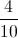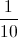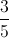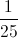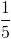Explanation:

Since there are 5 of each color, there are 25 total marbles in the bag. The probability of picking a red is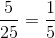### Example Question #1 : Ratio And Proportion

Ella has 6 cats and 36 dogs. What is the ratio of dogs to cats in Ella's house?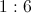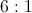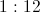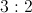Explanation:

There are 36 dogs and 6 cats, leaving a dog to cat ratio of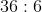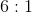.

### Example Question #5 : Ratio And Proportion

Sharon has 80 marbles, 60 of which are blue and 20 of which are white. Write the ratio of blue marbles to white marbles in simplest form.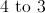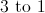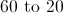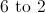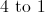Explanation:

The ratio of blue marbles to white marbles is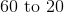, but this is not in simplest form. Rewrite this as a fraction for simplicity, then divide both numbers by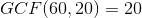: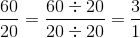In simplest form, the ratio is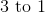### Example Question #6 : Ratio And Proportion

A class of 40 students has 25 boys and 15 girls. Write the ratio of boys to girls in simplest form.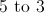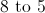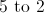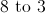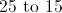Explanation:

The ratio of boys to girls is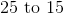, but this is not in simplest form. Rewrite this as a fraction for simplicity, then divide both numbers by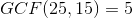: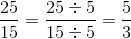In simplest form, the ratio is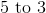### Example Question #7 : Ratio And Proportion

A total of 35 children took a poll about favorite breakfast foods, choosing between cereal, yogurt, and pancakes. If 14 preferred cereal and 7 preferred yogurt, how many preferred pancakes?

21

18

14

7

14

Explanation:

To find the children who preferred pancakes, subtract all the other children (cereal + yogurt) from the total: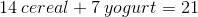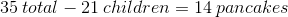### Example Question #8 : Ratio And Proportion

Susan has a bag of 12 assorted candies that included 3 blue, 2 green, 4 red, and 3 yellow. If Susan picks one piece of candy without looking, which color has a 1 in 6 chance of being picked?

green

yellow and blue

blue

yellow

red

green

Explanation:

There are 2 green candies in the assortment out of a total of 12 candies. The proportion of green candies to total candies is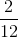, which simplifies to. This fraction describes a 1 in 6 chance, which is what we are looking for.

### Example Question #9 : Ratio And Proportion

Determine the ratio of 86 to 129.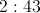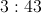Explanation:

Ratios represent how one number is related to another. These steps will help you determine the ratio of the numbers shown:

1) Divide both terms of the ratio by the GCF (greatest common factor). In this case, the GCF is 43 because 43 is the greatest number that goes into both numbers evenly.

2) Show the ratio with a colon : and remember to keep the numbers in the same order!

Therefore, the ratio of 86 to 129 is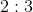.

### Example Question #10 : Ratio And Proportion

A truck with a tank that holds 24 gallons of gas gets 18 miles to the gallon. If the truck's gas gauge reads that the tank is one-half full, how many miles can it travel before it needs to be refueled?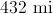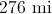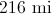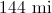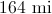Explanation:

Multiply 18 miles per gallon by 24 gallons to get the distance the truck can travel on a full tank: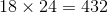miles

On one-half of a tank, this truck can travel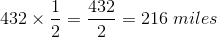← Previous 1 3 4 5 6